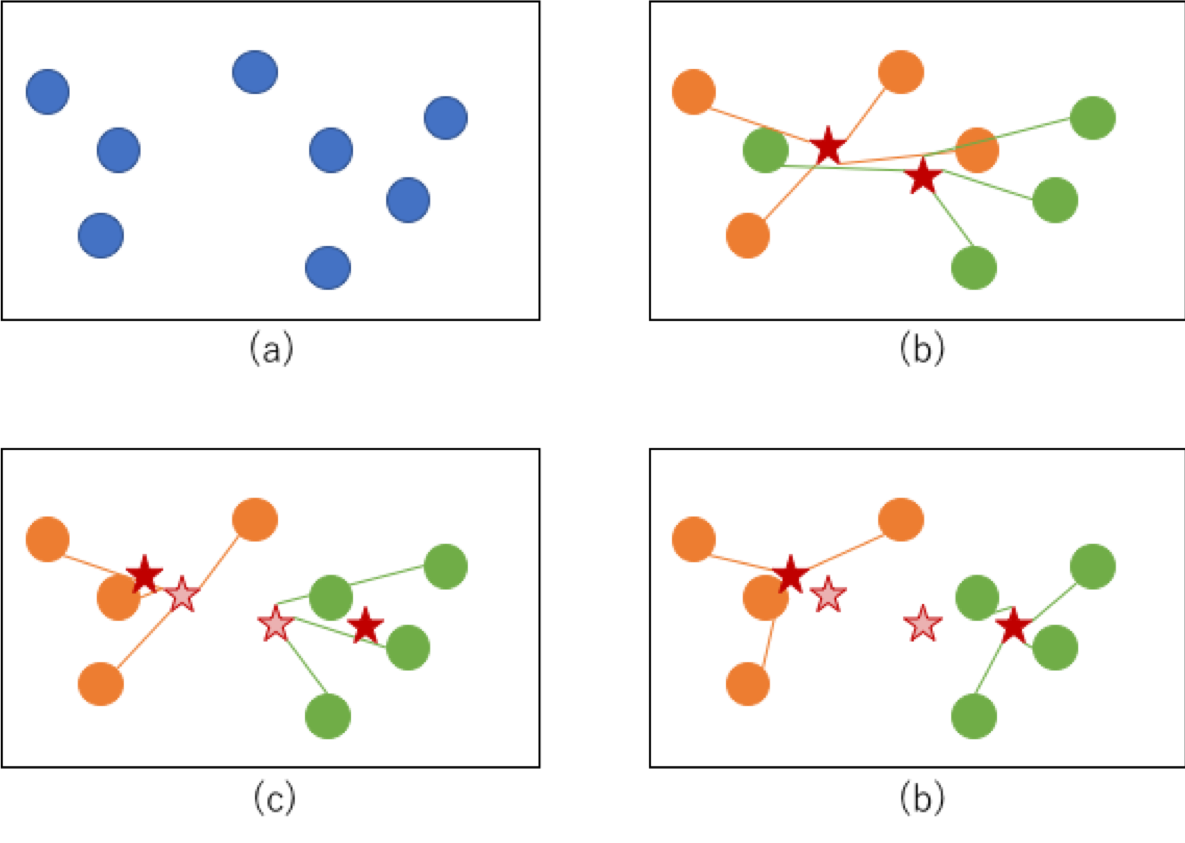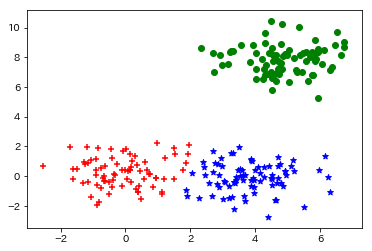# Introduction

I have summarized what I learned about the k-means method. This is the most basic clustering algorithm.

## reference

In understanding the k-means method, I referred to the following.

-Introduction to Machine Learning for Language Processing (Natural Language Processing Series) Daiya Takamura (Author), Manabu Okumura (Supervised) Publisher; Corona Publishing -Essence of Machine Learning Koichi Kato (Author) Publisher; SB Creative Co., Ltd.

# Outline of k-means method

## What is k-means method

The k-means method is an algorithm that first divides the data into appropriate clusters and then adjusts the data so that it is well divided using the average of the clusters. The k-means method (called the k-means method) because it is an algorithm that creates k clusters of arbitrary designation.

## k-means algorithm

Specifically, the k-means method follows the following steps.

1. Randomly allocate clusters for each point \$ x_ {i} \$
2. Calculate the centroid for the points assigned to each cluster
3. For each point, calculate the distance from the center of gravity calculated above and reassign it to the cluster with the closest distance.
4. Repeat steps 2 and 3 until the assigned cluster does not change.

Expressed in the figure, it is an image that the cluster converges in the order of (a) → (b) → (c) → (d) as shown below. At step (b), clusters are first assigned to each point and the center of gravity is calculated (the center of gravity is shown by the red star). In (c), the cluster is reassigned based on the distance from its center of gravity. (The new center of gravity is shown by the red star, the old center of gravity is shown by the thin red star). If this process is repeated and the cluster converges in a way that does not change as in (d), the process is complete.# Implement k-means method

## Implementation of k-means method without using library

The following is an implementation of the k-means method without using a library. Essence of machine learning A memo is written in the code.

``````import numpy as np
import itertools

class KMeans:
def __init__(self, n_clusters, max_iter = 1000, random_seed = 0):
self.n_clusters = n_clusters
self.max_iter = max_iter
self.random_state = np.random.RandomState(random_seed)

def fit(self, X):
#Generate a generator that repeatedly creates labels for the specified number of clusters (0),1,2,0,1,2,0,1,2...(Like)
cycle = itertools.cycle(range(self.n_clusters))
#Randomly assign a cluster label to each data point
self.labels_ = np.fromiter(itertools.islice(cycle, X.shape), dtype = np.int)
self.random_state.shuffle(self.labels_)
labels_prev = np.zeros(X.shape)
count = 0
self.cluster_centers_ = np.zeros((self.n_clusters, X.shape))

#Ends when the cluster to which each data point belongs does not change or exceeds a certain number of iterations
while (not (self.labels_ == labels_prev).all() and count < self.max_iter):
#Calculate the centroid of each cluster at that time
for i in range(self.n_clusters):
XX = X[self.labels_ == i, :]
self.cluster_centers_[i, :] = XX.mean(axis = 0)
#Brute force the distance between each data point and the center of gravity of each cluster
dist = ((X[:, :, np.newaxis] - self.cluster_centers_.T[np.newaxis, :, :]) ** 2).sum(axis = 1)
#Remember the previous cluster label. If the previous label and the label do not change, the program ends.
labels_prev = self.labels_
#As a result of recalculation, assign the label of the cluster closest to the distance.
self.labels_ = dist.argmin(axis = 1)
count += 1

def predict(self, X):
dist = ((X[:, :, np.newaxis] - self.cluster_centers_.T[np.newaxis, :, :]) ** 2).sum(axis = 1)
labels = dist.argmin(axis = 1)
return labels
``````

## Verification

The following is a verification of whether clustering is really possible with this algorithm.

``````import matplotlib.pyplot as plt

#Create a suitable dataset
np.random.seed(0)
points1 = np.random.randn(80, 2)
points2 = np.random.randn(80, 2) + np.array([4,0])
points3 = np.random.randn(80, 2) + np.array([5,8])

points = np.r_[points1, points2, points3]
np.random.shuffle(points)

#Create a model to divide into 3 clusters
model =  KMeans(3)
model.fit(points)

print(model.labels_)
``````

Then the output will look like this. You can see that the labels are brilliantly assigned to three.

``````[1 0 2 1 0 0 2 0 1 2 0 0 2 0 2 2 0 0 2 1 2 0 1 2 0 1 2 1 0 1 0 0 0 2 0 2 0
1 1 0 0 0 0 1 2 0 0 0 2 1 0 2 1 0 2 0 2 1 1 1 1 1 0 2 0 2 2 0 0 0 0 0 2 0
2 2 2 1 0 2 1 2 0 0 2 0 1 2 1 1 1 2 2 2 1 2 2 2 1 2 1 0 0 0 0 0 2 0 1 0 1
2 0 1 1 0 1 2 1 1 1 2 2 1 2 1 0 1 1 2 0 1 0 1 1 1 0 2 1 0 0 1 2 2 2 1 0 0
0 2 2 2 0 0 1 2 0 2 2 2 1 2 2 2 2 2 1 1 0 1 2 1 1 2 0 1 1 1 1 0 2 1 0 1 1
2 1 2 2 2 1 2 0 1 2 2 2 0 0 0 0 1 1 2 1 1 1 2 2 0 0 1 1 2 0 0 1 0 1 1 2 1
0 1 2 1 0 1 2 2 1 1 2 1 2 1 0 1 1 2]
``````

Let's illustrate this with matplotlib.

``````markers = ["+", "*", "o"]
color = ['r', 'b', 'g']
for i in range(3):
p = points[model.labels_ == i, :]
plt.scatter(p[:, 0], p[:, 1], marker = markers[i], color = color[i])

plt.show()
``````

Here is the output. You can see that clustering is completed without any problems.Next This k-means method has the problem that the accuracy changes depending on the allocation of the first random cluster. I would like to challenge the implementation of k-means ++, which is trying to overcome that problem.